# Covariance In ExcelArticle byExcelMojo Team## What Is Covariance Function In Excel?

The COVAR or the Covariance function in Excel calculates the relationship between two given datasets whether they vary together directly or inversely. Users can use the function to evaluate the relationship between two groups of values, such as analyzing whether, investing more in marketing results in improved sales. If the variables vary directly, it is a positive covariance, and if the variables vary inversely or in the opposite direction, it is a negative covariance.

The Covariance in Excel is replaced by COVARIANCE.P and COVARIANCE.S in Excel 2010. Thus, COVAR() is now available as a Compatibility function for backward compatibility.

For example, we must determine the covariance of two datasets given in the table below.

Select cell B13, enter the formula =COVAR(A2:A11,B2:B11), and enter the “Enter” key.

The result is ‘15.3’, as shown above.

[Output Observation: Mathematically, the COVAR() calculates the aggregate of the products of the deviations for every data point pair in the two given datasets and then averages the resulting sum value.

As the COVAR() output is positive, it indicates a positive correlation between the two sets of values. For example, if the first dataset’s values increase, the second also follows the same trend.]

###### Key Takeaways
• The COVAR function helps assess the correlation between two sets of values. For example, we can analyze the relationship between the revenue changes of two companies.
• The supplied arguments can be number arrays, named ranges, or cell range references to numbers.
• The function ignores the text, logical values, and blank cells in the two supplied arrays or cell ranges. However, it counts cells containing zero.
• Newer Statistical functions with improved accuracies, such as COVARIANCE.P and COVARIANCE.S, replaced the COVAR function in Excel 2010.

### COVAR() Excel Formula

The syntax of the COVAR Excel formula is,

The arguments of the COVAR Excel formula are,

• array1: The first set of integers or variables or cell range. It is a mandatory argument.
• array2: The second set of integers or variables or cell range. It is a mandatory argument.

### How To Calculate Covariance in Function?

We can use the COVAR Excel Function to calculate covariance in 2 ways, namely,

1. Access from the Excel ribbon.
2. Enter in the worksheet manually.

#### Method #1 – Access from the Excel ribbon

Choose an empty cell for output – select the “Formulas” tab – click the “More Functions” option drop-down – click the “Compatibility” option right arrow – select the “COVAR” function, as shown below.

The “Function Arguments” window appears. Enter the arguments in the “Array1”, and the “Array2” fields – click “OK”, as shown below.

#### Method #2 – Enter in the worksheet manually

1. Select an empty cell for the output.
2. Type =COVAR( in the cell. [Alternatively, type =C or =COV and double-click the COVAR function from the Excel suggestions.]
3. Enter the arguments as cell values or cell references and close the brackets.
4. Press Enter to execute and get the required covariance value.

Let us take a basic example to understand its calculations.

We will calculate the covariance of the two given arrays, which will help determine whether spending on marketing improves the company’s sales figures using Covariance in Excel.

The table below shows a small venture’s monthly marketing costs and sales data.

The steps to calculate Covariance in Excel using COVAR() are,

1. Select the target cell B15, and enter the formula =COVAR(B2:B13, i.e., the first variable range.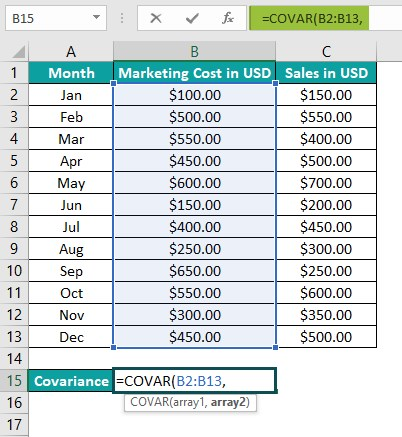2. Enter the second variable cell range as C2:C13, and close the brackets. The complete formula is =COVAR(B2:B13,C2:C13).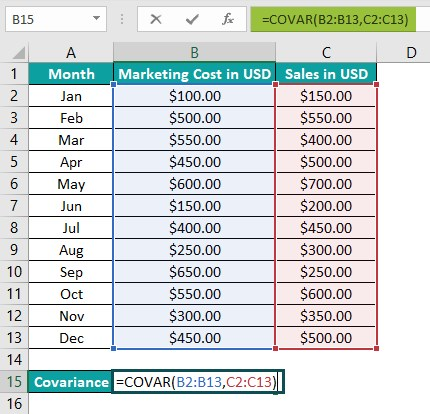3. Press the “Enter” key. The result is ‘18385.41667’, as shown below.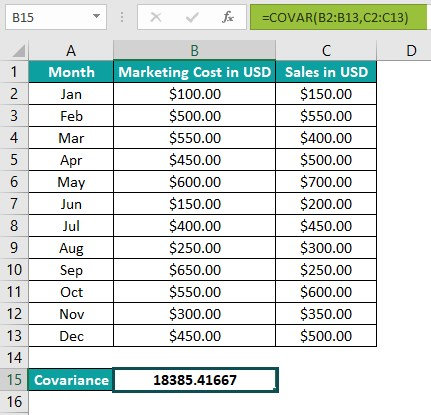[Alternatively, select the target cell B15 and go to FormulasMore FunctionsCompatibilityCOVAR to apply the function in the chosen cell.

The above step will open the Function Arguments window.

Next, enter the given cell ranges in the respective fields in the Function Arguments window.

Finally, click OK to close the window, and we will see the COVAR() output in the target cell B15.]

[Output Observation: In the above example, the COVAR() accepts the two given array ranges as its arguments, B2:B13 and C2:C13. It then determines the average of the products of deviations for every data value pair in the two arrays and returns the covariance as 18385.41667.

And since the COVAR() output is positive, it indicates a positive correlation between marketing costs and sales figures. Therefore, it implies that spending more on marketing improves the company’s sales.]

### Examples

We will see some advanced scenarios using the Covariance in Excel examples.

#### Example #1

We will calculate the covariance between the two sets of given data using the Covariance function.

The below table shows the 10-day daily returns data of two stocks in a portfolio.

The procedure to calculate the covariance using the COVAR() is,

Select the target cell B13, enter the formula =COVAR(B2:B11,C2:C11), and press Enter.

The result is ‘-0.59’, as shown above.

[Output Observation: The result shows a negative correlation between the two stock sets. Thus, suggesting that if one stock shows a positive daily returns trend, the other will have a negative trend.]

#### Example #2

Mathematically, the below equation represents Covariance in Excel.

The terms and ȳ denote the array1, and array2 mean values. And  is the given sample’s size.

We shall see how the above equation works and gives the same result as the Covariance function in the following example to determine the covariance of the two given arrays.

The first table contains the two given datasets, Group 1 and Group 2, containing numbers.

Assume the first array is x and the second one is y. And the sample size n is 10 (The number of data points in each dataset).

The steps to calculate the covariance using COVAR() using AVERAGE Function are,

Step 1: Select cell D4, enter the formula =AVERAGE(A3:A12) to get the first array’s mean value, and press Enter. The result is ‘53.4’, as shown below.

Step 2: Select cell E4, enter the formula =AVERAGE(B3:B12) to get the second array’s mean value, and press Enter. The result is ‘10’, as shown below.

Step 3: Select cell G4, enter the formula=A3-\$D\$4 to determine the deviation of the first data point in Group 1 from its mean, and press Enter. The result is ‘-9.5’, as shown below.

Next, drag the formula from cell G4 to G13 using the fill handle to iterate the process for all the data points in Group 1. The output is shown below.

Step 4: Select cell H4, enter the formula =B3-\$E\$4 to determine the deviation of the first data point in Group 2 from its mean, and press Enter. The result is ’91.8’, as shown below.

Next, drag the formula from cell H4 to H13 using the fill handle to iterate the process for all the data points in Group 2. The output is shown below.

Step 5: Select cell I4, enter the formula =G4*H4 to find the product of deviation of the first data point pair in the two given datasets, and press Enter.

Next, drag the formula from cell I4 to I13 using the fill handle to iterate the process of all the data point pairs in the two groups. The output is shown below.

Step 6: Select the target cell K3, enter the formula =SUM(I4:I13)/D7 representing the mathematical expression of Covariance, and press Enter. The result is ‘25’, as shown below in cell K3.

First, the cell K3 formula determines the aggregate of the products of the deviations we found in cells I4:I13. It then finds the average of the resulting sum value, with the sample size n being 10.

Step 7: Select the target cell L3, enter the formula =COVAR(A3:A12,B3:B12) to find the covariance of the two given datasets, and press Enter. The result is ‘25’, as shown below in cell L3.

[Output Observation: The above example shows the covariance value obtained by solving the mathematical expression in cell K3 and applying the COVAR() in cell L3 is the same.]

#### Example #3

In the following example, we will compare the Covariance in Excel, i.e., COVAR(), and the latest Statistical functions that replaced it to determine the covariance of the two firms’ revenues.

While one new function is the COVARIANCE.P(), which gives the Population Covariance, the other is COVARIANCE.S(), returns the Sample Covariance in Excel.

The first table shows the yearly revenue data of Firm A and Firm B.

The steps to calculate the covariance and compare the results with COVAR(), COVARIANCE.P(), and COVARIANCE.S() are,

Step 1: Select cell E3, enter the COVAR() formula =COVAR(B3:B9,C3:C9), and press Enter.

Step 2: Select cell F3, enter the COVARIANCE.P() formula =COVARIANCE.P(B3:B9,C3:C9), and press Enter.

Step 3: Select cell G3, enter the COVARIANCE.S() formula =COVARIANCE.S(B3:B9,C3:C9), and press Enter.

The results are shown above in cells E3, F3, and G3.

[Output Observation: All three functions accept the cell range references to the two given datasets. While the COVAR() and COVARIANCE.P() return the result 3.918367347, the COVARIANCE.S() returns the Sample Covariance in Excel as 4.571428571.

The difference in the function outputs is because the mathematical formula for COVAR() and COVARIANCE.P() is the same. However, in the case of the COVARIANCE.S(), the denominator term in the corresponding mathematical expression is n-1 instead of n, where n is the sample size.

In the above example, the determined covariance is positive, which indicates a positive correlation between the two firms’ revenues.]

### Important Things To Note

• The COVAR() returns the #N/A error if the two supplied arrays are of different sizes.
• If we provide empty datasets or datasets with no numbers as the arguments, the COVAR function output will be the #DIV/0! error.
• We must ensure the two given arrays contain numbers. They should not be empty, and the standard deviation of their data points should not be zero.

1. How does the function Covariance in Excel, COVAR, work when the input arrays also contain text values along with numbers?

The COVAR works in the following way when the input arrays contain text values with numbers.

Let us see the steps with an example.

The below table shows two datasets containing numbers and text values.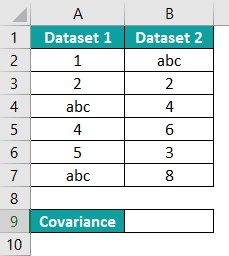The procedure to find the covariance using the COVAR() is,
Select the target cell B9, enter the formula =COVAR(A2:A7,B2:B7), and press Enter.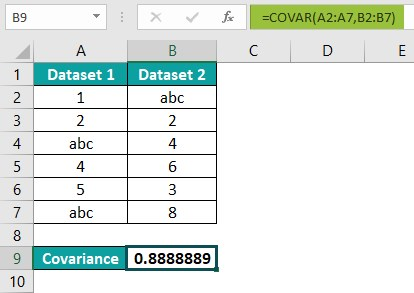The result is ‘0.888889’, as shown below.

[Output Observation: The COVAR() accepts the references to the two given data ranges and returns their covariance as 0.888889. The function ignores the text values and counts only the numbers to calculate the required covariance.]

2. How to apply the Covariance in Excel VBA?

We can apply the Covariance and Excel VBA by applying the following method:
But if we require to use the latest Covariance functions, apply the below methods in Excel VBA.
The two arguments in the above methods are the same as those explained in the COVAR() Excel Formula section.

3. Why is the COVAR function not working in Excel?

The COVAR function may not work for the following reasons:
One of the supplied arrays does not contain any numbers.
One of the supplied arrays is empty.
The selected datasets or cell ranges are of different sizes.